Next: Implementation Up: Interval Analysis Previous: Interval Analysis   Contents

Mathematical background

This section is freely inspired from the book . An interval number is a real, closed interval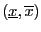. Arithmetic rules exist for interval numbers. For example let two interval numbers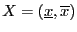,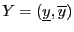, then: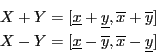An interval function is an interval-valued function of one or more interval arguments. An interval function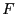is said to be inclusion monotonic if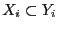for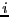in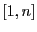implies: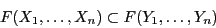A fundamental theorem is that any rational interval function evaluated with a fixed sequence of operations involving only addition, subtraction, multiplication and division is inclusion monotonic. This means in practice that the interval evaluation of a function gives bounds (very often overestimated) for the value of the function: for any specific values of the unknowns within their range the value of the function for these values will be included in the interval evaluation of the function. A very interesting point is that the above statement will be true even taking into account numerical errors. For example the number 1/3, which has no exact representation in a computer, will be represented by an interval (whose bounds are the highest floating point number less than 1/3 and the smallest the lowest floating point number greater than 1/3) in such way that the multiplication of this interval by 3 will include the value 1. A straightforward consequence is that if the interval evaluation of a function does not include 0, then there is no root of the function within the ranges for the unknowns.

In all the following sections an interval for the variable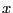will be denoted by. The width or diameter of an intervalis the positive difference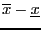. The mid-point of an interval is defined as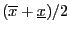.

A box is a set of intervals. The width of a box is the largest width of the intervals in the set and the center of the box is the vector constituted with the mid-point of all the intervals in the set.Next: Implementation Up: Interval Analysis Previous: Interval Analysis   Contents
Jean-Pierre Merlet 2012-12-20